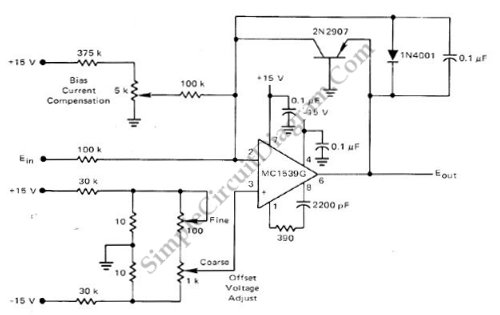# Low-Cost Logarithmic Converter Using Opamp and Transistor

This low-cost logarithmic converter is built using op-amp and transistor. This circuit uses a Motorola MC1539G op-amp which is connected to PNP transistor. To compensate the error, this circuit need an external compensation which can accommodates wide range of input voltage and has about 200nA bias current. To adjust bias current initially, replace the transistor with 500K potentiometer and get the gain of 5 over the input signal by adjusting the 5K pot. Here is the schematic diagram of the circuit:[Circuit’s schematic diagram source: Motorola Application Notes]